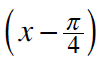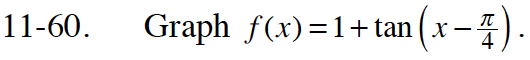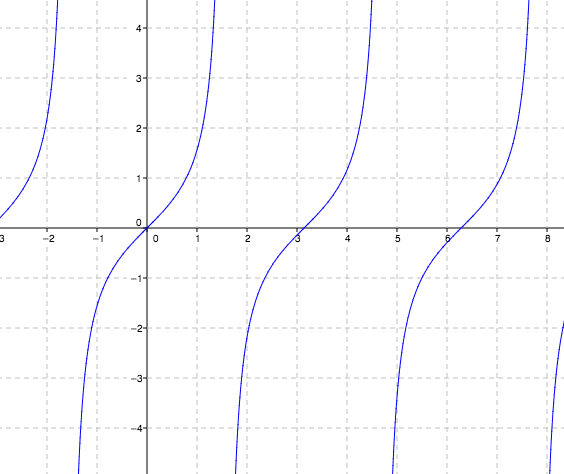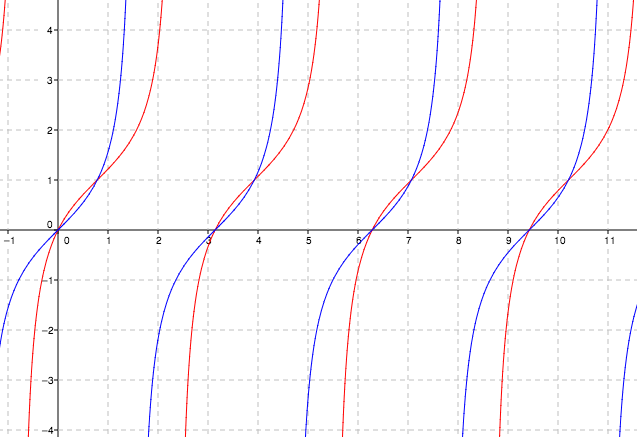Home > INT3 > Chapter 11 > Lesson 11.2.1 > Problem11-60

11-60.

Graph f(x) = 1 + tan. Homework Help ✎Here is the original graph of f(x) = tan(x).How does adding 1 shift the graph?

$\text{How does subtracting }\footnotesize \frac{\pi }{4} \text{ from \textit{x}\, shift the graph?}$